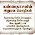### Previous GATE Questions on Multiplexers (MUX) with Solutions (1987 - Till Date)

1992

1.       The logic realized by the circuit shown in figure is

2000

1.   A one bit full adder is to be implemented using 8 to 1 multiplexers (MUX).
a.       Write the truth table for sum (S) and carry to the next stage (CN), in terms of the two bits (A, B) and the carry from the previous stage (CP). The truth table should be in the ascending order of (A, B, CP) i.e. (000, 001, 010..... etc).
b.      Implement S and CN using 8 to 1 multiplexers.

2001

1.       In the TTL circuit in the figure, S2 to S0 are select lines and X7 to X0 are input lines. S0 and X0 are LSBs. The output Y is

2002

1.       The inputs  to a digital circuit shown in figure is are the external signals A, B and C. Assume complements of A, B and C are not available. The +5 volts power supply (logic 1) and the ground (logic 0) are also available.
a.       Write down the output function in its canonical SOP and POS forms.
b.      Implement the circuit using only 2 to 1 multiplexers shown in the figure, where S is the data select line, D0 and D1 are the input data lines and Y is the output line. The function table is also given for the multiplexer.

2003

1.       Without any additional circuitry, an 8:1 MUX can be used to obtain
a.       Some but not all Boolean functions of 3 variables
b.      All functions of 3 variables but none of 4 variables
c.       All functions of 3 variables and some but not all of 4 variables
d.      All functions of 4 variables

2004

1.       The minimum number of 2 to 1 multiplexers required to realize a 4 to 1 multiplexer is
a.       1
b.      2
c.       3
d.      4

2005

1.       The Boolean function f implemented in the figure using two input multiplexers is

2007

1. In the following circuit X is given by

2008

1.       For the circuit shown in the following figure, I0 – I3 are inputs to the 4:1 multiplexer, R(MSB) and S are control inputs. The output Z can be represented by

2009

1.       What are the minimum number of 2 to 1 multiplexers required to generated a 2 input AND gate and a 2 input EX-OR gate?
a.       1 and 2
b.      1 and 3
c.       1 and 1
d.      2 and 2

2010

1.       The Boolean function realized by the logic circuit shown is

a.       F = m(0,1,3,5,9,10,14)
b.      F = m(2,3,5,7,8,12,13)
c.       F = m(1,2,4,5,11,14,15)
d.      F = m(2,3,5,7,8,9,12)

2011

1.       The logic function implemented by the circuit below is (ground implies a logic ‘0’)

a.       F = AND (P,Q)
b.      F = OR (P,Q)
c.       F = XNOR (P,Q)
d.      F = XOR (P,Q)

2014
1.       Consider the multiplexer based logic circuit shown in the figure

2.       In the circuit shown, W and Y are MSBs of the control inputs. The output F is given by

3.       If X and Y are inputs and the Difference (D = X – Y) and the Borrow (B) are the outputs, which one of the following diagrams implements a half-subtractor?

4.       An 8 – to – 1 multiplexer is used to implement a logical function Y as shown in the figure. The output Y is given by

1.super questions

2.nice explations..
thnks

3.4.Thanks for this explanation

1.our pleasure

5.good content.

in question 1, A and B are missing and option 2 should be A exor B.

Name

Email *

Message *